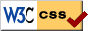Welcome to A!Die Software Studio

# C++ 运算符优先级列表

by other
2011-05-13 10:31:29

## C++ Operator Precedence

The operators at the top of this list are evaluated first. Operators within a group have the same precedence. All operators have left-to-right associativity unless otherwise noted.

1 `::` scope resolution no left to right
2 `()` function call yes left to right
`[]` array access yes
`->` member access yes
`.` no
`++  --` postfix yes
`dynamic_cast  static_cast  reinterpret_cast  const_cast` type conversion no
`typeid` Get type information no
3 `!  not` logical negation yes right to left
`~  compl` bitwise negation (complement) yes
`++  --` prefix yes
`+  -` unary sign operations yes
`*  &` indirection and reference yes
`sizeof` Size (of the type) of the operand in bytes no
`new  new[]  delete  delete[]` dynamic memory management yes
`(type)` Cast to a given type yes
4 `->*` member pointer selector yes left to right
`.*` member object selector no
5 `*`  `/`  `%` arithmetic operations yes left to right
6 `+`  `-`
7 `<<  >>` shift operations yes left to right
8 `<  <=  >  >=` relational operations yes left to right
9 `==  != not_eq`
10 `&  bitand` bitwise AND yes left to right
11 `^  xor` bitwise XOR yes left to right
12 `|  bitor` bitwise OR yes left to right
13 `&&  and` logical AND yes left to right
14 `||  or` logical OR yes left to right
15 `?:` Ternary conditional (if-then-else) no right to left
16 `=  +=  -=  *=  /=  %=  &=  ^= |= <<= >>=` assignment yes right to left
17 `,` Sequential evaluation operator yes left to right

## Order of Evaluation and of Side Effects

One important aspect of C++ that is related to operator precedence, is the order of evaluation and the order of side effects in expressions. In most circumstances, the order in which things happen is not specified. For example in `f() + g()` whether `f()` or `g()` is called first is not specified.

Further, the effect of certain expressions is undefined. For example, consider the following code:

`    float x = 1;     x = x / ++x;`

The value of x and the rest of the behavior of the program after evaluating this expression is undefined. The program is semantically ill-formed: x is modified twice between two consecutive sequence points. For identification of sequence points, see Annex C of the C ISOStandard.

Expressions like the one above must be avoided. When in doubt, break a large expression into multiple statements to ensure that the order of evaluation is correct.

To determine the order of execution of operators consider these rules in the given order:

1) Consider effects of precedence-changing tokens: parentheses () and brackets []

2) Inherent precedence of operator.

3) When 1) above does not apply and two operators have the same precedence consider the operator associativity. For example, << associates left to right which means that the left-operand is the first operand, and = associates right to left. Note then that stream operators execute left to right while the expression a = b = c evaluates right to left.

### ▲评论

X 正在回复:

Ｑ Ｑ: